# How to calculate the equivalent resistance in this Circuit?

VitaminK
Homework Statement:
Calculate the equivalent resistance. Is it possible to calculate an exact value?
Relevant Equations:
Series: R1+R2+R3....Rn = Req
Parallel: (1/Req)=(1/R1)+(1/R2)…(1/Rn)
I start from far right:
Green and blue are parallel Connection: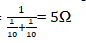This in turn is in series connection with black resistor: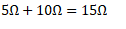and so on ...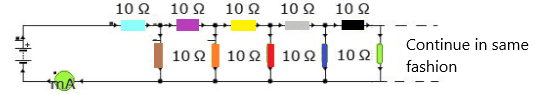This is what I'm ending up withMy final equivalent resistance is (275/17) Ω. Is this correct?

Gold Member
Homework Statement:: Calculate the equivalent resistance. Is it possible to calculate an exact value?
Relevant Equations:: Series: R1+R2+R3...Rn = Req
Parallel: (1/Req)=(1/R1)+(1/R2)…(1/Rn)

Green and blue are parallel Connection
What about the black resistor? Either this was a simple oversight, or you need to review what parallel resistors are.

Gold Member
Also, take note that this circuit goes on forever to the right side. There is no "right most" resistor. You said you started at the right; I think you started somewhere in the middle.

VitaminK
Oh right, I get it now. So I will never be able to calculate an exact value because the Circuit is never ending. Maybe I should start from the left and work my way up to at least five more parallel resitors just to show that.

Homework Helper
Gold Member
Oh right, I get it now. So I will never be able to calculate an exact value because the Circuit is never ending. Maybe I should start from the left and work my way up to at least five more parallel resitors just to show that.
Actually, you can calculate the exact value. There is a trick to getting the answer that might be hard to see if you've never seen the trick before! It's also hard to give a hint without giving the whole thing away.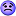You are interested in the total resistance of the network shown in figure (a) below: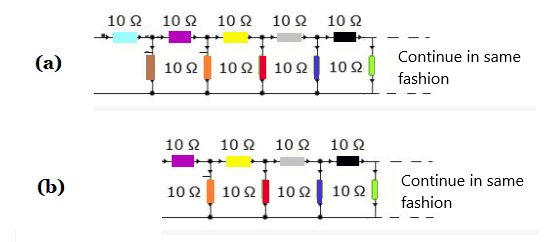Consider the part of this network that is to the right of the brown resistor, as shown in (b).

Gold Member
You can model all of the stuff to the right of some arbitrary point as a single resistor of (initially) unknown value. Then look for patterns. It's sort of like the infinite series calculations that you may have seen in your math classes.

VitaminK
What about the black resistor? Either this was a simple oversight, or you need to review what parallel resistors are.
Actually, you can calculate the exact value. There is a trick to getting the answer that might be hard to see if you've never seen the trick before! It's also hard to give a hint without giving the whole thing away.You are interested in the total resistance of the network shown in figure (a) below:
View attachment 258264

Consider the part of this network that is to the right of the brown resistor, as shown in (b).

Actually, you can calculate the exact value. There is a trick to getting the answer that might be hard to see if you've never seen the trick before! It's also hard to give a hint without giving the whole thing away.You are interested in the total resistance of the network shown in figure (a) below:
View attachment 258264

Consider the part of this network that is to the right of the brown resistor, as shown in (b).

The equivalent resistance for orange and red:The equivalent resistance for 5Ω-resistor and Purple:
5Ω+10Ω = 15Ω

The equivalent resistance for 15Ω-resistor and blue: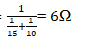The equivalent resistance for 6Ω-resistor and yellow:
6Ω+10Ω=16Ω

The equivalent resistance for 16Ω-resistor and green: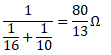The equivalent resistance for 80/13Ω-resistor and gray: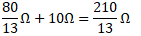The equivalent resistance for 210/13Ω-resistor and black: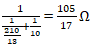If this calculation is correct , do you mean that this pattern will repeat itself?

Homework Helper
Gold Member
The equivalent resistance for orange and red:
View attachment 258269

As @DaveE pointed out earlier, the orange and red resistors are not in parallel due to the yellow resistor between them. So, you cannot combine them to get 5Ω equivalence.

Going back to the picture in post #5, how does the total resistance of figure (b) compare to the total resistance of figure (a)?

•scottdave
Homework Helper
Gold Member
It is sort of like this puzzle:
$$x = 1+ \frac { 1 } { 1 + \frac {1} {1 + ...}}$$
Can you find the value of ##x## ?
If you can figure how to do that one, then the same type of thinking can be applied to the circuit network.

•TSny
VitaminK
It is sort of like this puzzle:
$$x = 1+ \frac { 1 } { 1 + \frac {1} {1 + ...}}$$
Can you find the value of ##x## ?
If you can figure how to do that one, then the same type of thinking can be applied to the circuit network.

less than 2?
As @DaveE pointed out earlier, the orange and red resistors are not in parallel due to the yellow resistor between them. So, you cannot combine them to get 5Ω equivalence.

Going back to the picture in post #5, how does the total resistance of figure (b) compare to the total resistance of figure (a)?

I guess the total resistance in figure b is less than a?

VitaminK
As @DaveE pointed out earlier, the orange and red resistors are not in parallel due to the yellow resistor between them. So, you cannot combine them to get 5Ω equivalence.

Going back to the picture in post #5, how does the total resistance of figure (b) compare to the total resistance of figure (a)?

Then purple must be in series with orange, yellow with red, grey with blue and finally black with green, or is it wrong?

Homework Helper
Gold Member
I guess the total resistance in figure b is less than a?
Can you find any difference in the networks of (a) and (b)? [Other than the colors!]

Homework Helper
Gold Member
Then purple must be in series with orange, yellow with red, grey with blue and finally black with green, or is it wrong?
None of the individual resistors in the network are in series or parallel.

Gold Member
Perhaps it would help if you first solve the finite circuit that only has the left-most 4 resistors. Then solve it for the left-most 6 resistors, then 8 ... Look for a pattern. How does adding 2 more resistors to the previously solved version change the answer?

Homework Helper
Gold Member
Less than 2 is a good guess. I really don't want to give it away @VitaminK . If you can arrive at the method of solving with just a little prompting, it will help your problem solving skills, because you'll probably remember it better.
$$x = 1+ \frac { 1 } { 1 + \frac {1} {1 + ...}}$$

So look at just the denominator of the first 1. How would you compare that expression to the whole expression?

•hutchphd
VitaminK
Less than 2 is a good guess. I really don't want to give it away @VitaminK . If you can arrive at the method of solving with just a little prompting, it will help your problem solving skills, because you'll probably remember it better.
$$x = 1+ \frac { 1 } { 1 + \frac {1} {1 + ...}}$$

So look at just the denominator of the first 1. How would you compare that expression to the whole expression?
The expression in the denominator is the same as the whole expression?

•scottdave
Homework Helper
Gold Member
The expression in the denominator is the same as the whole expression?
So using that information, you could solve for an actual value of x.

Now how can you apply that thinking to the resistors. Is there a portion of the whole network, which looks like the whole network. Then we can go about solving for the value of "X" resistance.

VitaminK
Can you find any difference in the networks of (a) and (b)? [Other than the colors!]
two resistors less

VitaminK
So using that information, you could solve for an actual value of x.

Now how can you apply that thinking to the resistors. Is there a portion of the whole network, which looks like the whole network. Then we can go about solving for the value of "X" resistance.

I guess that would be the resistors in b)

Homework Helper
Gold Member
I guess that would be the resistors in b)
Yes

VitaminK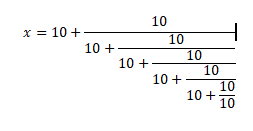something like this?

Homework Helper
Gold Member
If R is the unknown equivalent resistance of (a), what is the equivalent resistance of (b)?

VitaminK
It's R

Homework Helper
Gold Member
It's R
Yes. Can you redraw network (a) so that it takes this into account and becomes a simpler network?

VitaminK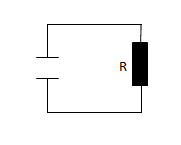like this?

Homework Helper
Gold Member
Well, this is a correct drawing. But it is not what I had in mind. Make use of the fact that (a) and (b) have the same resistance R.

•scottdave
VitaminK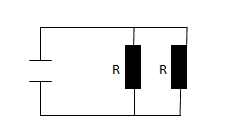Homework Helper
Gold Member
No. This is not correct. It will probably help if you explain in words how you got this incorrect diagram.

VitaminK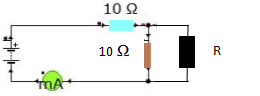I've now replaced circuit b) with R.

Homework Helper
Gold Member
View attachment 258304I've now replaced circuit b) with R.
So find an expression for the resistance of this circuit (leaving in the variable R). Then, as you figured out, this circuit has the same resistance as the one you drew in post #25.

VitaminK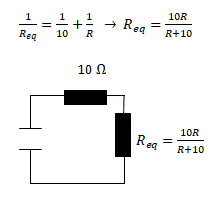is this the expression?

Homework Helper
Gold Member
View attachment 258309is this the expression?
Yes. Good. But keep going. Can you continue to simplify this circuit by combining the two resistors in your diagram?

VitaminK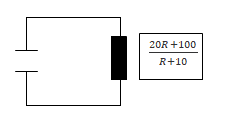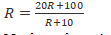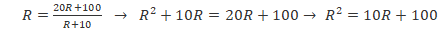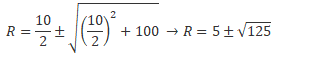Think I got it now. Thanks everyone!

•scottdave and TSny
Homework Helper
Gold Member
Only one of those values is possible in a real circuit since one is positive and the other is negative.
Now that you solved it, here's a check for your answer. Let's start several nodes out to the right.
We have the 10Ω in parallel with the unknown equivalent resistance to the right of that. Let's call that R0.

If we move 1 node to the left, then R1 = 10Ω + (10Ω)(R0) / ( 10Ω + R0) . This is the Product / Sum for parallel resistances. You can keep going: R2 = 10Ω + (10Ω)(R1) / ( 10Ω + R1) and so on.

So why am I doing all of this? You can iterate till it converges on a number. Even though we don't know what R0 is, we can take an initial guess. We know it should be larger than 10Ω, so pick 11 as an initial guess.

I used Excel. Put my guess for R0 in the first cell {A1}, then in A2 I put the following formula: =10+10*A1/(10+A1)

I copied this down several rows. You can see that it converges fairly fast to a number (numerically close to your answer).

But even if I put wild guesses like 0 or 1 million, it still converges pretty close after only 5 or 6 iterations.